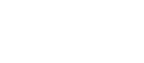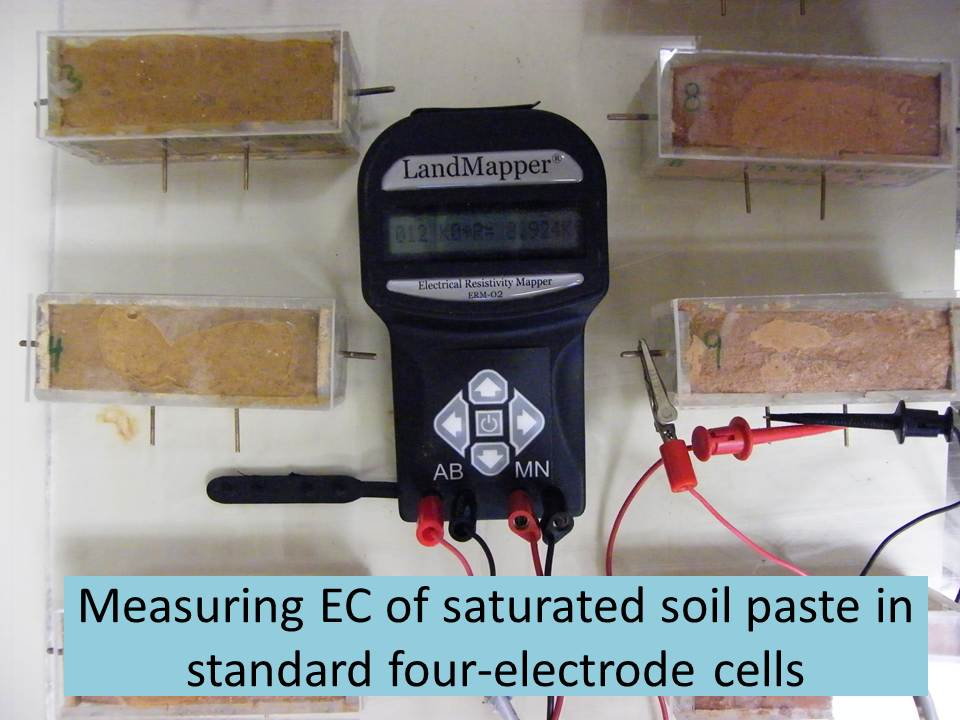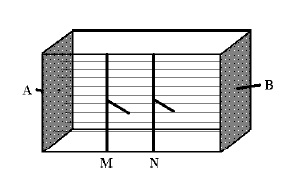Ландвайзер, LLC Ученье - свет...
 Поиск на сайте:

# Calibrate (calculate) K-coefficient for laboratory four-electrode cellLaboratory cells supplied by Landviser, LLC have been calibrated and the respective K-geometric coefficient is printed on the cell. The cells have to be filled to the top rim, in order for the coefficient to be accurate. Also, sometimes due to corrosion of conductive plates (electrodes) the coefficient of the cell might change slightly. Thus, you can verify K-coefficient of any cell, just follow instructions below.

For other tips, download Measuring Properties of Natural Systems with LandMapper ERM-02 (manual)

Materials:Fig. Scheme of the four-electrode laboratory conductivity cell. Electrical field lines are shown with thin straight lines (uniform electrical field).
1. Laboratory cell with embedded four-electrodes
2. Distilled water and standard resistivity solution (f.e. from Fisher Scientific, Inc.)
3. LandMapper with four banana plugs to alligator clips connectors.

Procedure:

1. Connect LandMapper to electrodes embedded in the cell. Landviser’s supplied rectangular cells have current (AB) electrodes as metal plates at the end of rectangular prism and potential (MN) electrodes as wire lines embedded on the front. Connect LandMapper terminals accordingly.
2. Fill cell with standard solution (ERss=X) with known resistivity. Measure ER on K0=1.
3. Record measured resistivity ERm=Y.
4. Measured ER times cell K-coefficient should be equal ERss:

Therefore, K of the cell is equal ERss divided by ERm: